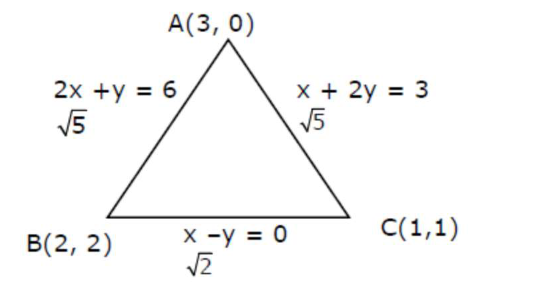# The intersection of three linesQuestion:

The intersection of three lines $x-y=0, x+2 y=3$ and $2 x+y=6$ is a:

1. (1) Equilateral triangle

2. (2) None of the above

3. (3) Isosceles triangle

4. (4) Right angled triangle

Correct Option: , 3

Solution: